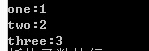#include
using namespace std;
class num
{
public:
num(){n=1;cout<<"构造函数执行\n";}
num(int i){n=i;cout<<"带一个参数的构造函数执行\n";}
num(const num&s){this->n=s.n;cout<<"复制构造函数执行\n";}
~num(){cout<<"析构函数执行\n";}
int get()const{return n;}
void set(int x){n=x;}
const num operator+(const num&r){return num(n+r.get());}

private:
int n;
};
int main()
{
num one(1),two(2),three;
three=one+two;
cout<<"one:"<<one.get()<<endl;
cout<<"two:"<<two.get()<<endl;
cout<<"three:"<<three.get<<endl;
return 0;

}

3个回答anhaoxuan 谢谢您的指点 这些日子我忙于其他事物现在才采纳 抱歉 愿您幸福平安！
4 年多之前 回复

three.get()